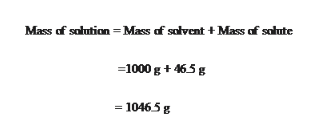# An aqueous solution of glucose (MM = 180.2 g/mol) has a molality of 5.21 m and a density of 1.20 g/mL. What is the molarity of glucose in the solution?

Question
718 views

An aqueous solution of glucose (MM = 180.2 g/mol) has a molality of 5.21 m and a density of 1.20 g/mL. What is the molarity of glucose in the solution?

check_circle

Step 1

Given:

Molality of Glucose = 0.258 m

Density of glucose solution = 1.20 g/mL

First calculate the mole of Glucose as follows:

Let, we assume that the mass of solution is 1.0kg

Step 2

To find the Weight of Glucose:

Step 3

To find the Mass o...help_outlineImage TranscriptioncloseMass of solution Mass af salvent Mass of salute 1000 g 465g -1046.5 g fullscreen

### Want to see the full answer?

See Solution

#### Want to see this answer and more?

Solutions are written by subject experts who are available 24/7. Questions are typically answered within 1 hour.*

See Solution
*Response times may vary by subject and question.
Tagged in

### Inorganic Chemistry